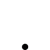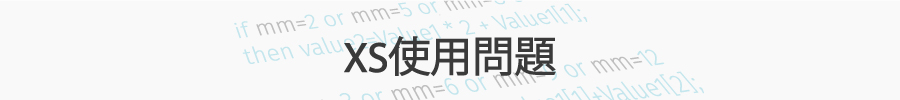請問我的做空策略寫法哪裡有問題

•   109
• 最後發表   查理布朗  2018 七月 16

input: Length1(3),Length2(6),Length3(12),Length4(48),Length5(60);

settotalbar(maxlist(Length5,6) * 13 * 7 + 8);
if barfreq ="Min" and Time > 084500 and Time <= 133000 then

value1 = ema(close,Length1);
value2 = ema(close,Length2);
value3 = ema(close,Length3);
value4 = directionmovement(Length3, value5, value6, value7);
value8 = ema(close,Length5);

condition1 = value1 crosses below value8;
condition2 = value8 <= value8[Length3];
condition3 = value5 < value6 and value5 < value5[Length1] and value6 > value6[Length1];

if condition2 and condition3 then ret = condition1;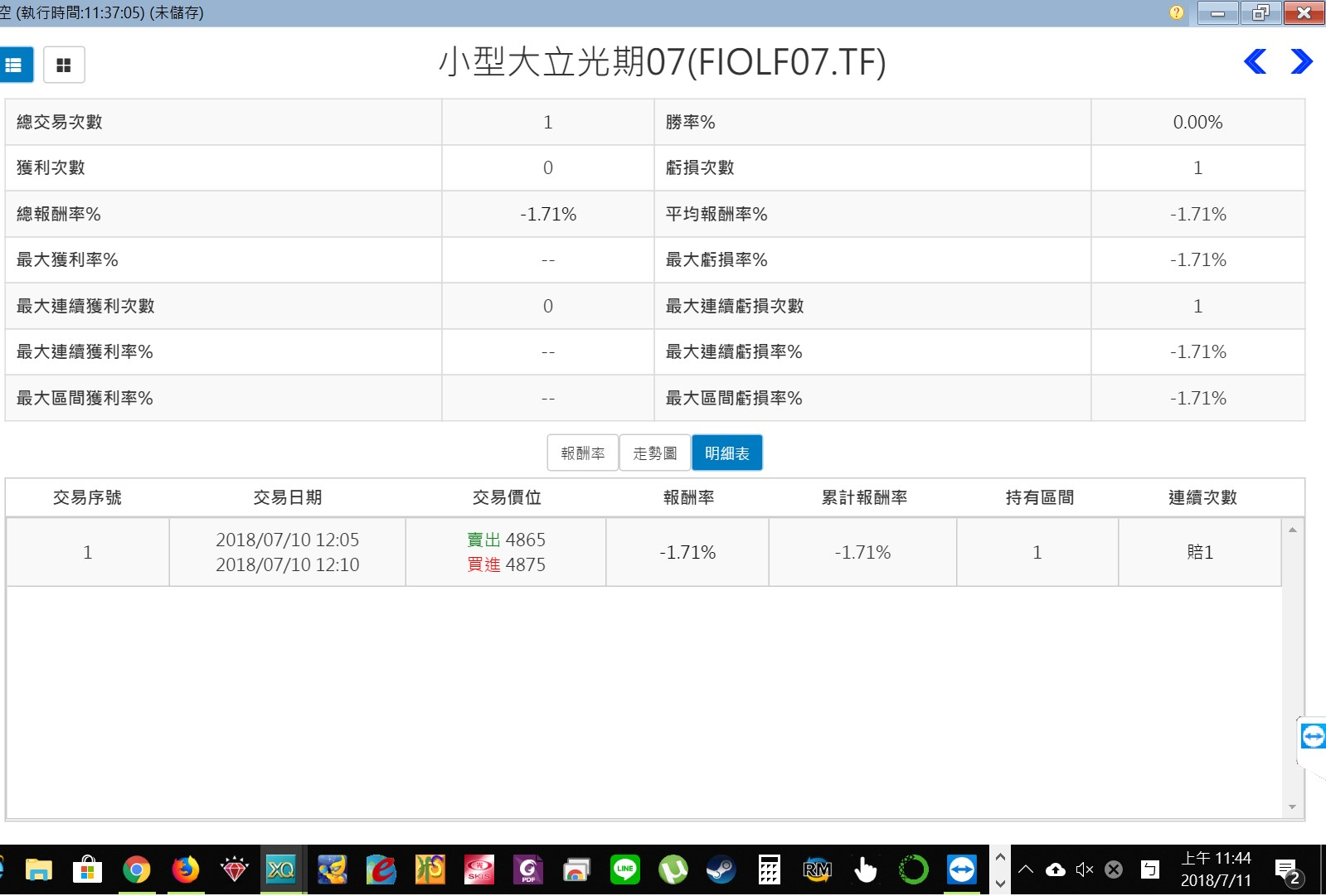XQ小幫手 發文於   2018/07/12

Hi 查理布朗,

XQ小幫手 發文於   2018/07/16

Hi 查理布朗,

XQ小幫手 發文於   2018/07/16

Hi 查理布朗,

+DI大於前一個+DI，-DI小於前一個-DI，+DI大於-DI

directionmovement(Length3, value5, value6, value7);

- 第二個參數(value5)是輸出計算完的+DI值。

- 第三個參數(value6)是輸出計算完的-DI值。

```condition3 = value5 > value6    //+DI大於-DI
and value5 > value5   //+DI大於前一個+DI
and value6 < value6;  //-DI小於前一個-DI```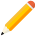發表新主題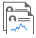我的發文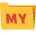我的收藏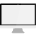分類

Close